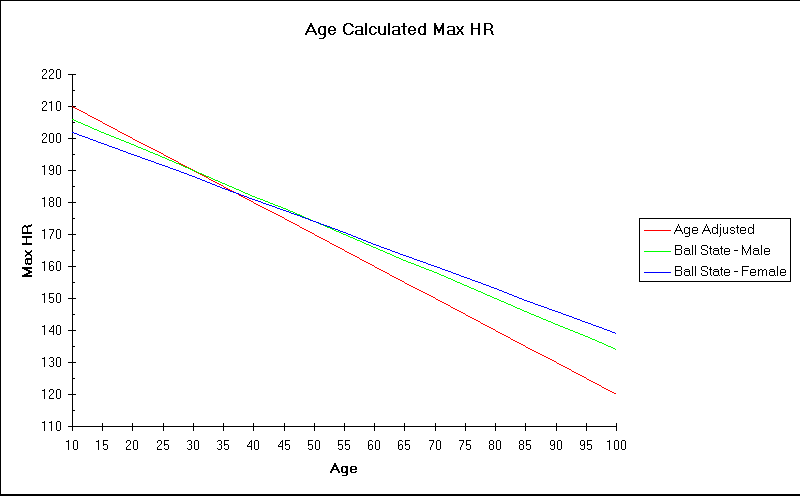# Heart Rate Training Formulae

 Home Training Zones & my Adjustment to Karvonen The Sums Site Info

### Calculating Maximum Heart Rate (MHR)

```1. The standard age derived method which is

MHR = 220 - age       (for me 220 - 51 = 169).

2. The Ball State University Method
Regarded as more accurate for the youngest and 'older' people:

For Men    MHR = 214 - (0.8 * age)
For Women  MHR = 209 - (0.7 * age)

```

The following graph indicates the discrepancy between the two methods:### Calculating Training Heart Rates (THR)

Each zone has a lower and upper Training Heart Rate (THR) - repeated here.

```Moderate Training Zone  = 50 - 60%  of MHR
Weight Management Zone  = 60 - 70%  of MHR
Aerobic Training Zone   = 70 - 80%  of MHR
Anaerobic Training Zone = 80 - 90%  of MHR
Red Line Training Zone  = 90 - 100% of MHR

I include three methods of calculating the limits of the zones.
For each of these the work rate ratio is the percentage divided by 100 i.e.
For 50% use 0.5, for 60% use 0.6 etc repeat the sum up to 90%.

1. Max HR method - Multiply MHR by the work rate ratio.
THR = MHR * Work Rate Ratio.

2. The Karvonen method that uses one's Resting Heart Rate.
a) Calculate Heart Rate Reserve; HRR = MHR - RHR and use this in the formula
b) THR = RHR + (HRR * work rate ratio)

3. My adjusted Karvonen method that reduces the RHR by multiplying it by 0.7;
this gives results between the other two methods.
a) Calculate Adjusted Rest Heart Rate; ARHR = RHR * 0.7
b) Calculate Heart Rate Reserve; HRR = MHR - ARHR and use this in the formula
c) THR = ARHR + (HRR * work rate ratio)

```

You don't need to do these sums - go to The Calculators.

The following graph indicates the discrepancy between the three methods:The area around 50% to 60% of effort is the practical range where the difference is most noticeable.